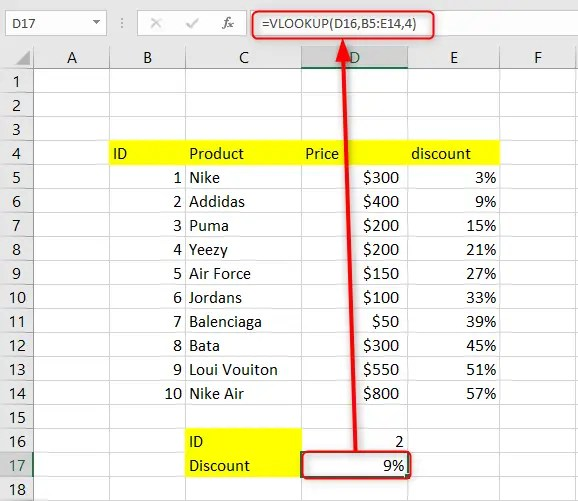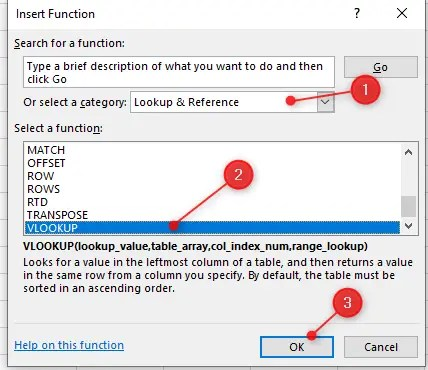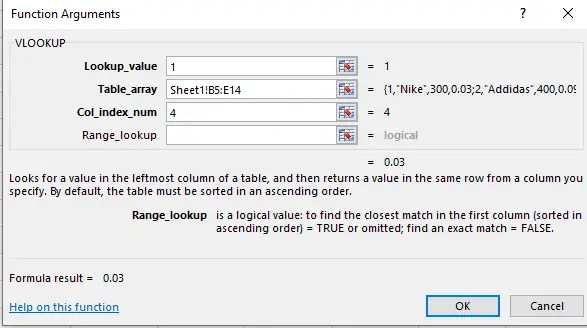# How to vlookup in Excel from another cell

VLOOKUP is one of Excel’s most useful functions. It is also the most misunderstood function, as people find it hard to apply. This function looks up a value in the first column of a given range and returns a value in the same row of another column. In short, this function will search through your data set based on the unique identifier and brings you any piece of information or data associated with your unique identifier.

In this article, we make the VLOOKUP function easy to understand. We give you a step-by-step explanation of how to vlookup in Excel from another cell.

## Using the VLOOKUP formula to vlookup in Excel from another cell

Here you will use the formula;

`=VLOOKUP (lookup_value, sheet! Range, column, match)`

The formula can also be written as

`=VLOOKUP (lookup_value, table_array, col_index_num, [range_lookup])`

whereby:

Lookup_value represents the value you want to search for.

Table_array represents the range of cells where you want to search for the lookup value and where you will retrieve a match. It is important to note that the VLOOKUP function will always search in your data’s first column of the table array. It searches in the leftmost column of your table array and returns a value from a column to the right.

Col_index_num represents the number of columns from which to return a value.

Range_lookup is an optional argument that determines whether to search for an approximate or exact match. It can present a TRUE or omitted result or FALSE, which is the exact match.For example, in the screenshot above, we have used the formula =VLOOKUP(D16,B5:E14,4) to lookup the value of the discount of a product based on its ID.

VLOOKUP practice sheet

## How to vlookup from another sheet in Excel

In your formula, you will put the worksheet’s name followed by an exclamation mark in your table_array argument. You will do this before the range reference. For example, let’s say you want to search in the range A2:C15 on sheet 3. Your formula will be like this:

`=VLOOKUP(1,Sheet1!B5:E14,4)`

1. In an open Excel worksheet, click on an empty cell.

2. Start by typing the formula in an empty cell where you want your formula to be calculated and the results displayed.

3. When it comes to typing in your table_array argument, switch to the worksheet you want to look up in and select the range of cells with your mouse.

4. Click the Enter key.5. Apart from this, you can click on the Formulas tab in the main menu ribbon.

6. In the Function Library group, click on the Lookup & Reference option.

7. In the displayed dropdown list, click on the option VLOOKUP which will be at the bottom of the list to display a dialog window.8. In the display Function Arguments window, specify your cells. You will need to enter your value or reference your cells in the Lookup-value field, Table_array field, Col_index_num field, and Range_lookup field.

9. Remember the Range_lookup in option. If you want an exact match, enter the FALSE option and if you want an approximate match, enter the TRUE option.10. Click the OK button at the bottom of the dialog window to apply the formula.